# NCERT Exemplar Solutions Class 9 Mathematics Chapter 12 Heron's Formula

## NCERT Exemplar Solutions Class 9 Maths Chapter 12 – Free PDF Download

NCERT Exemplar Class 9 Maths Chapter 12 Heron’s Formula, provided here in PDF format so that students can easily download it and prepare for the final exam. All the solutions are provided by our subject experts with respect to CBSE latest syllabus (2021-2022). With the help of these materials, students can revise the chapter and score good marks in the exam. The topics covered in Chapter 12 for Exemplar problems and solutions are:

• Area of a triangle based on its base and heights
• Area of a triangle by Heron’s Formula
• Application of Heron’s Formula in finding areas of Quadrilaterals

In geometry, the name Herons Formula named after Hero of Alexandria. In this chapter, the students will learn to calculate the area of a triangle using Heron’s Formula. In order to understand the chapter in a better way, students are advised to solve the maximum number of questions from  NCERT Exemplar Class 9 Maths Chapter 12 Heron’s Formula.

## Download PDF of NCERT Exemplar Solutions for Class 9 Maths Chapter 12 Heron’s Formula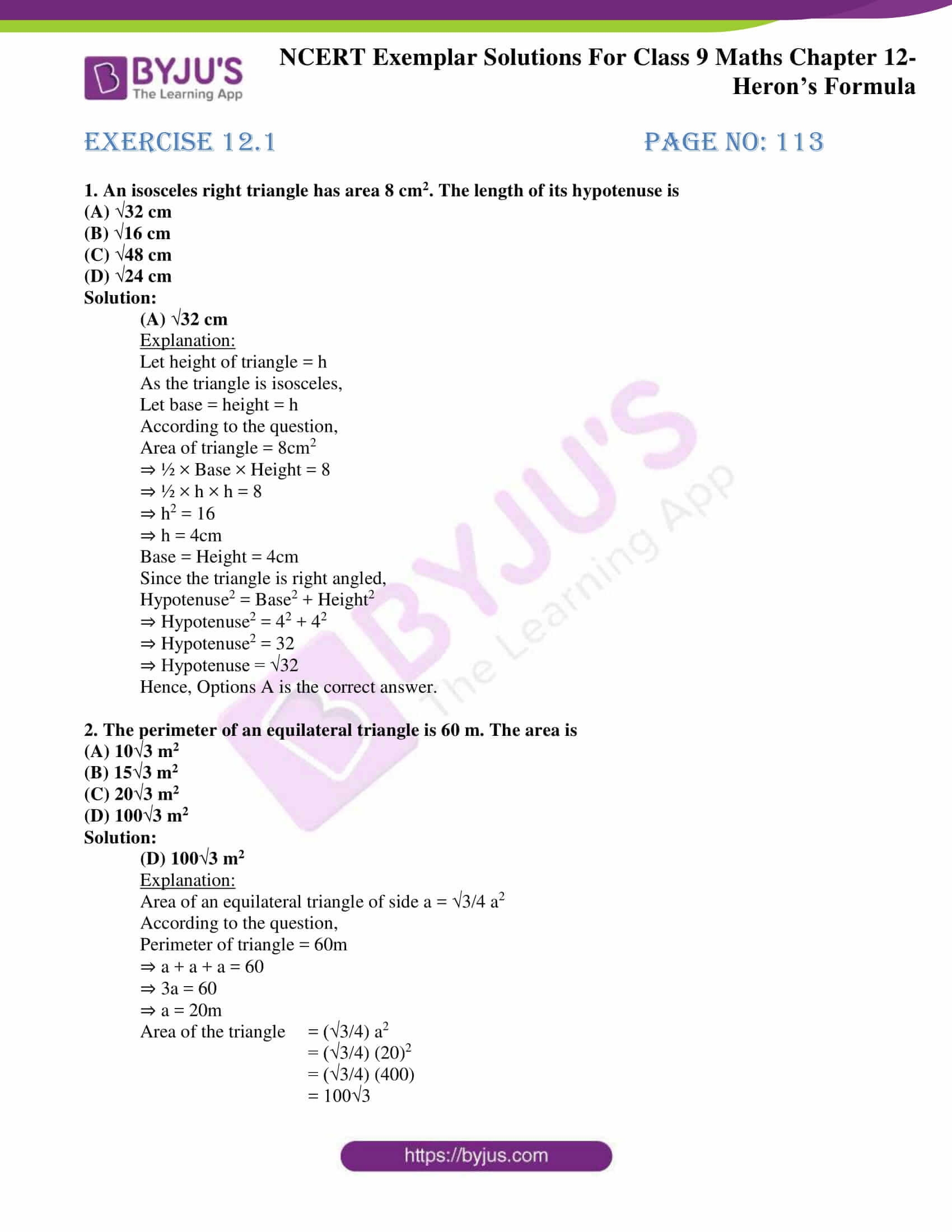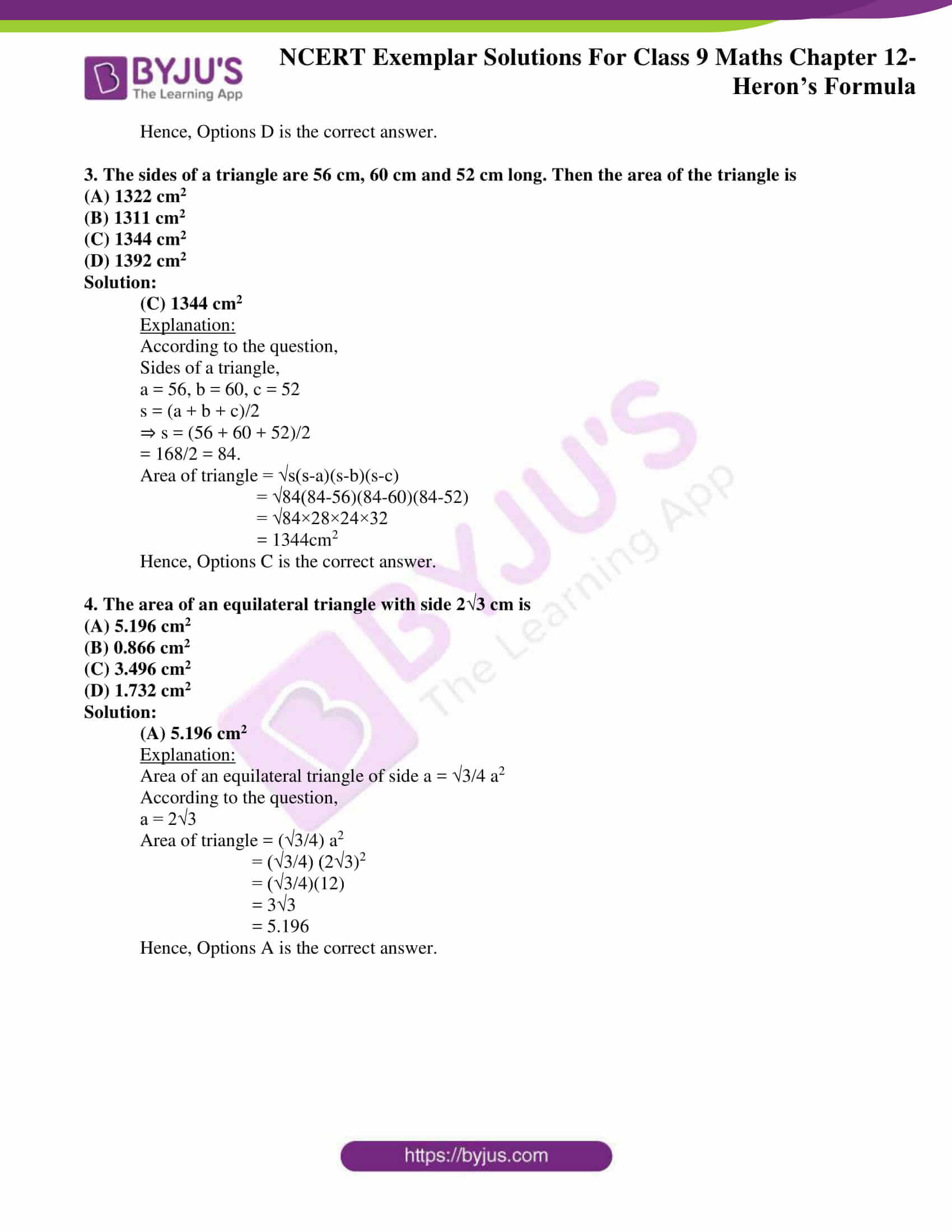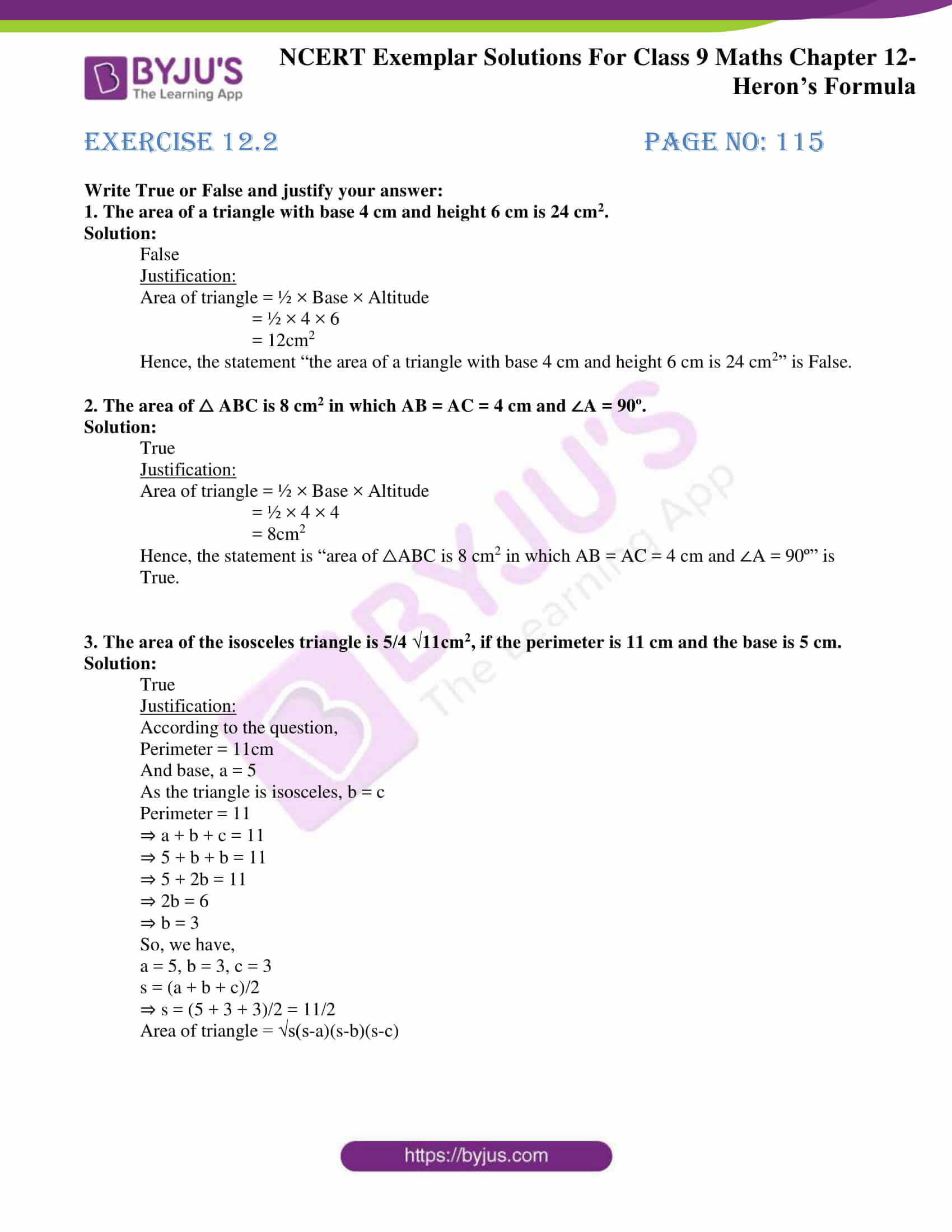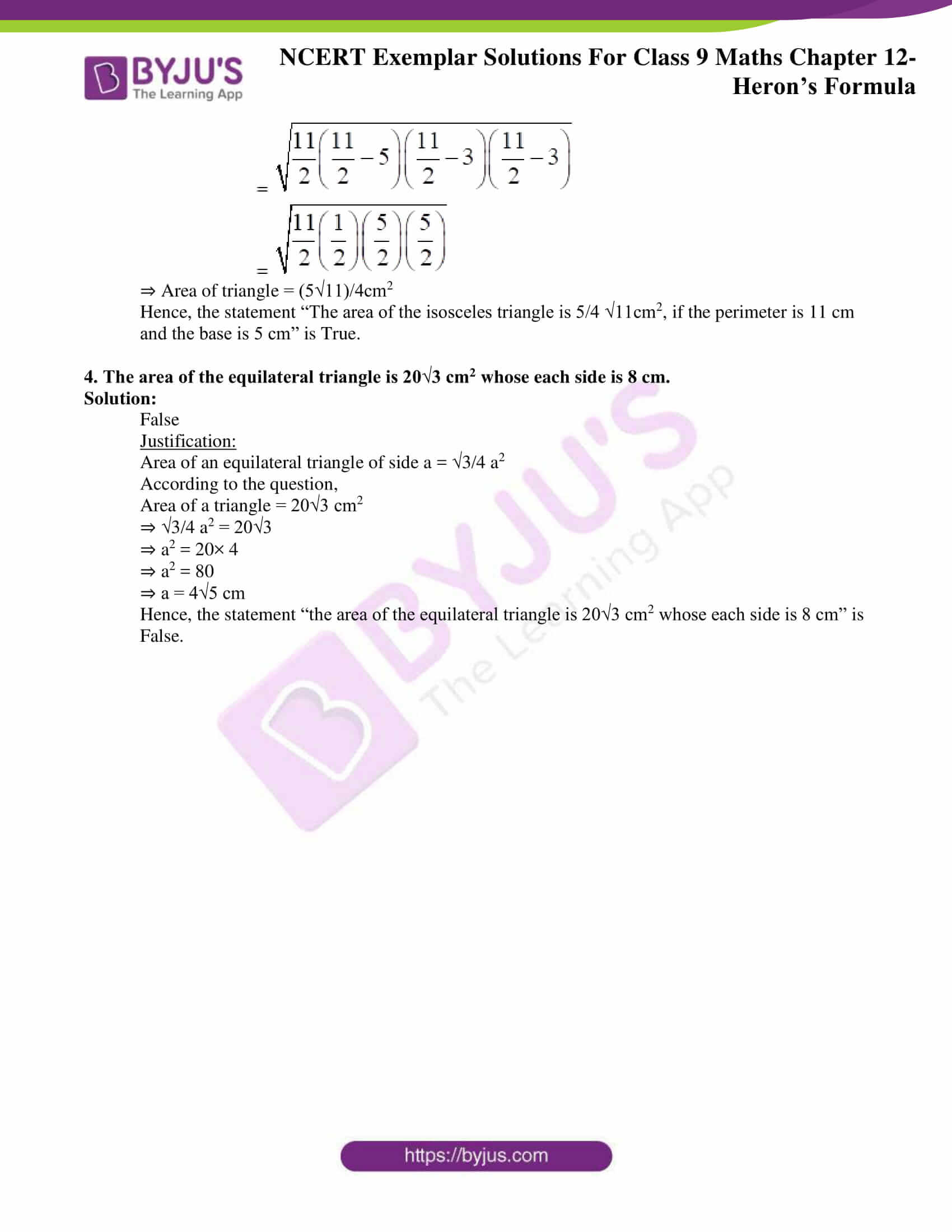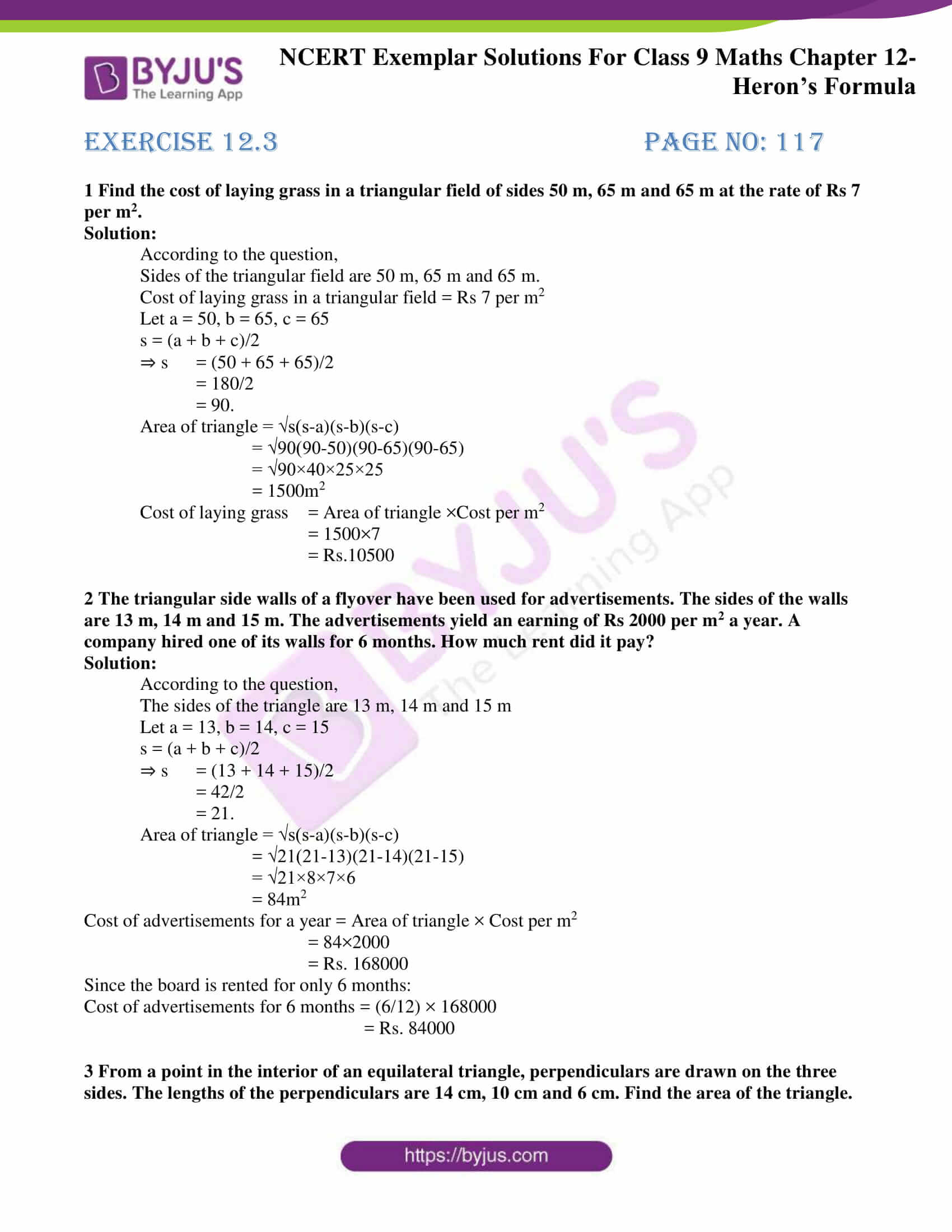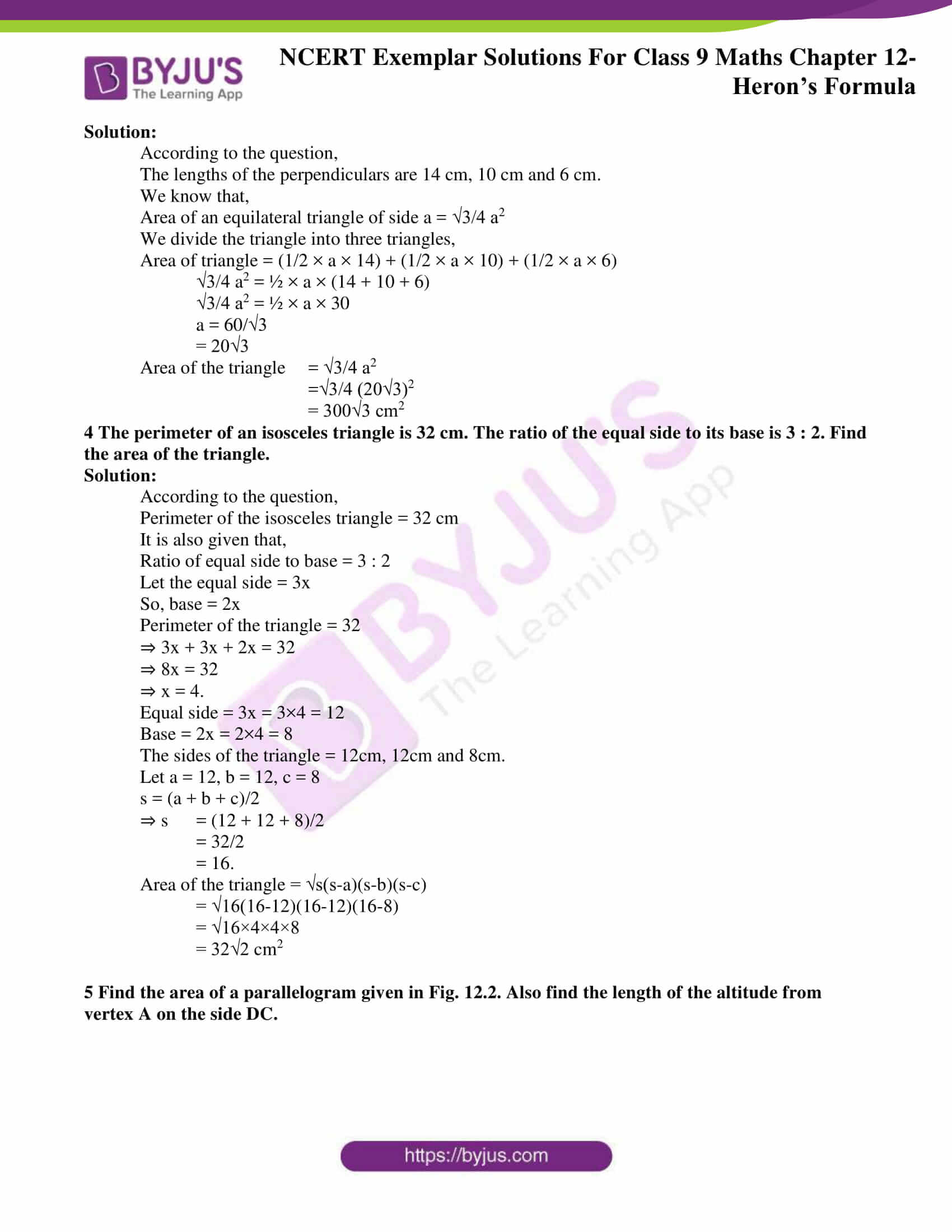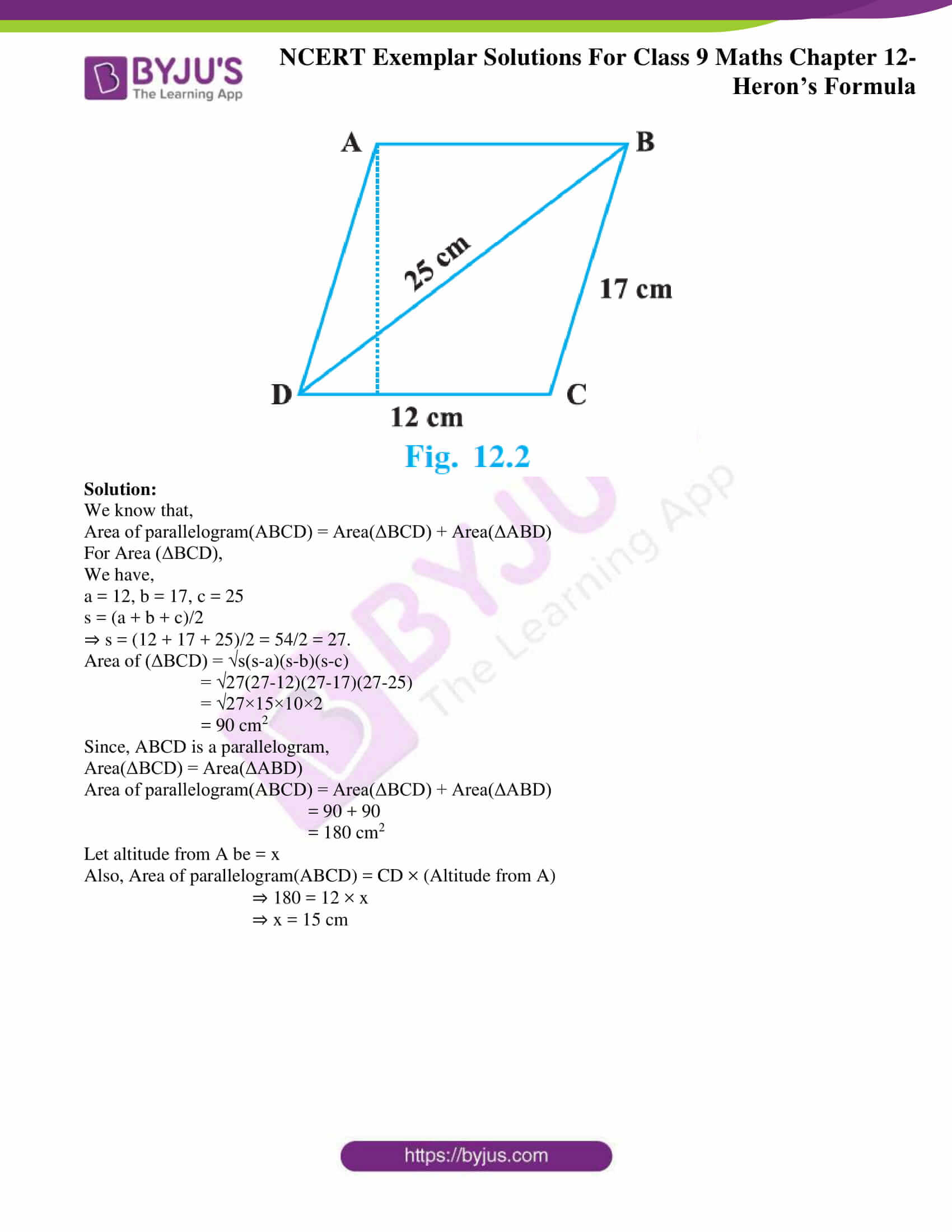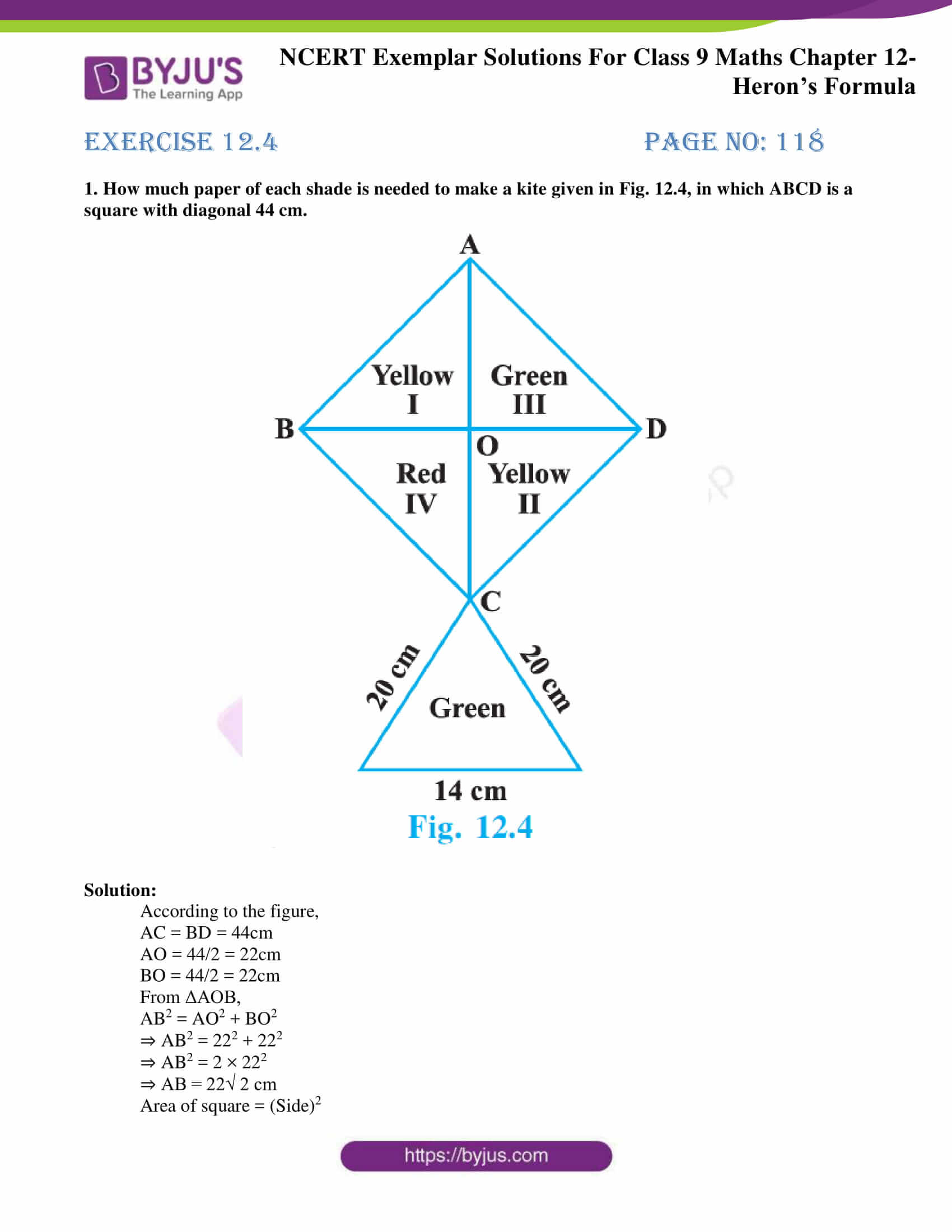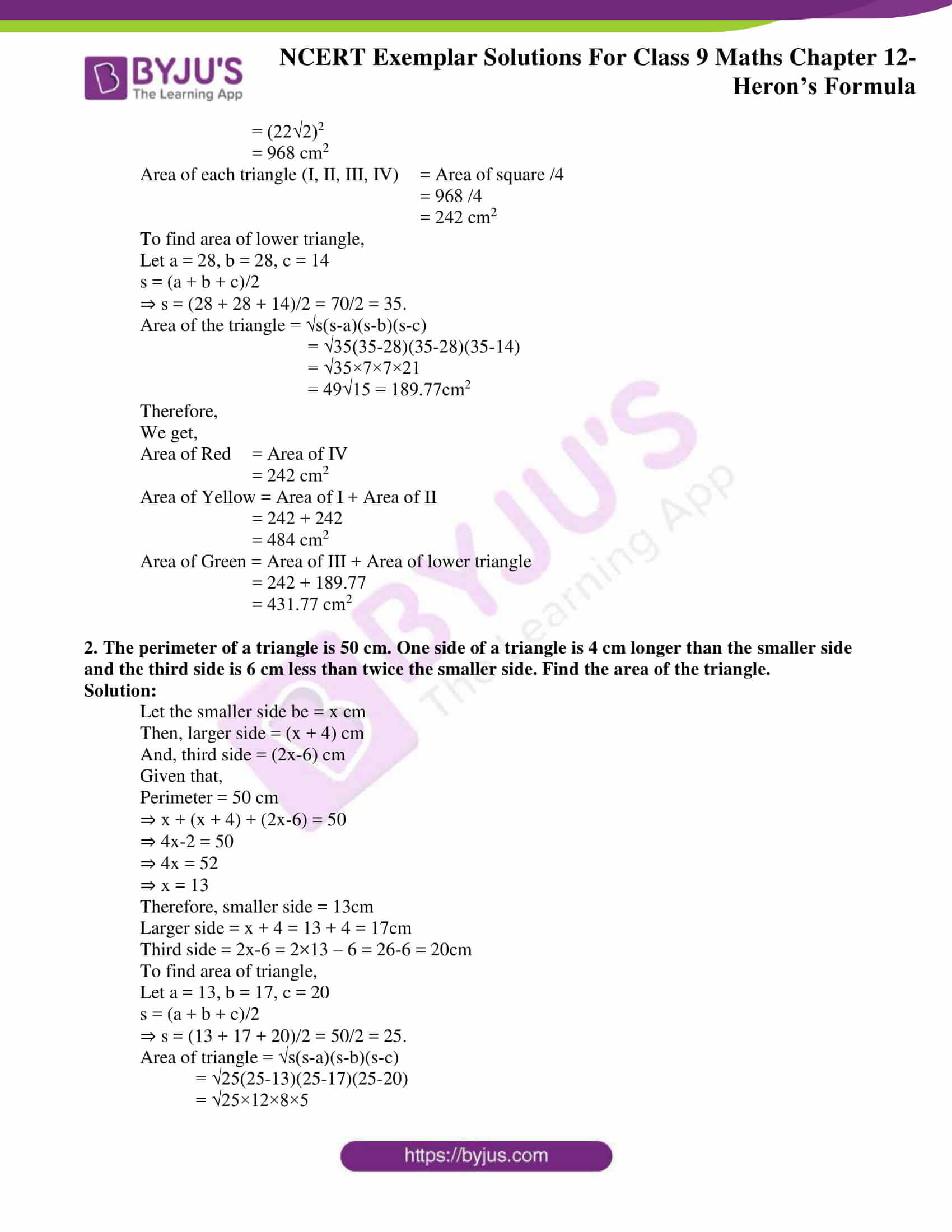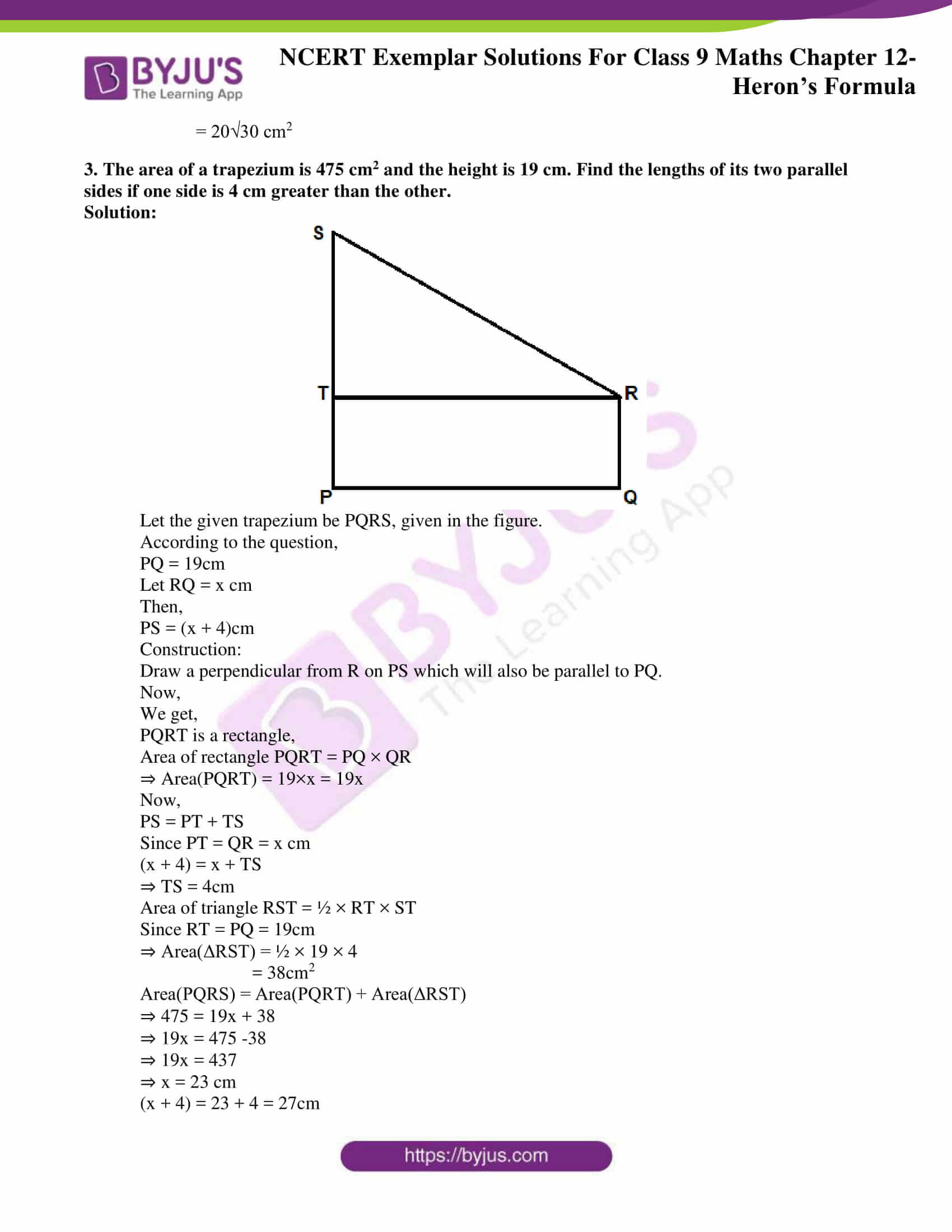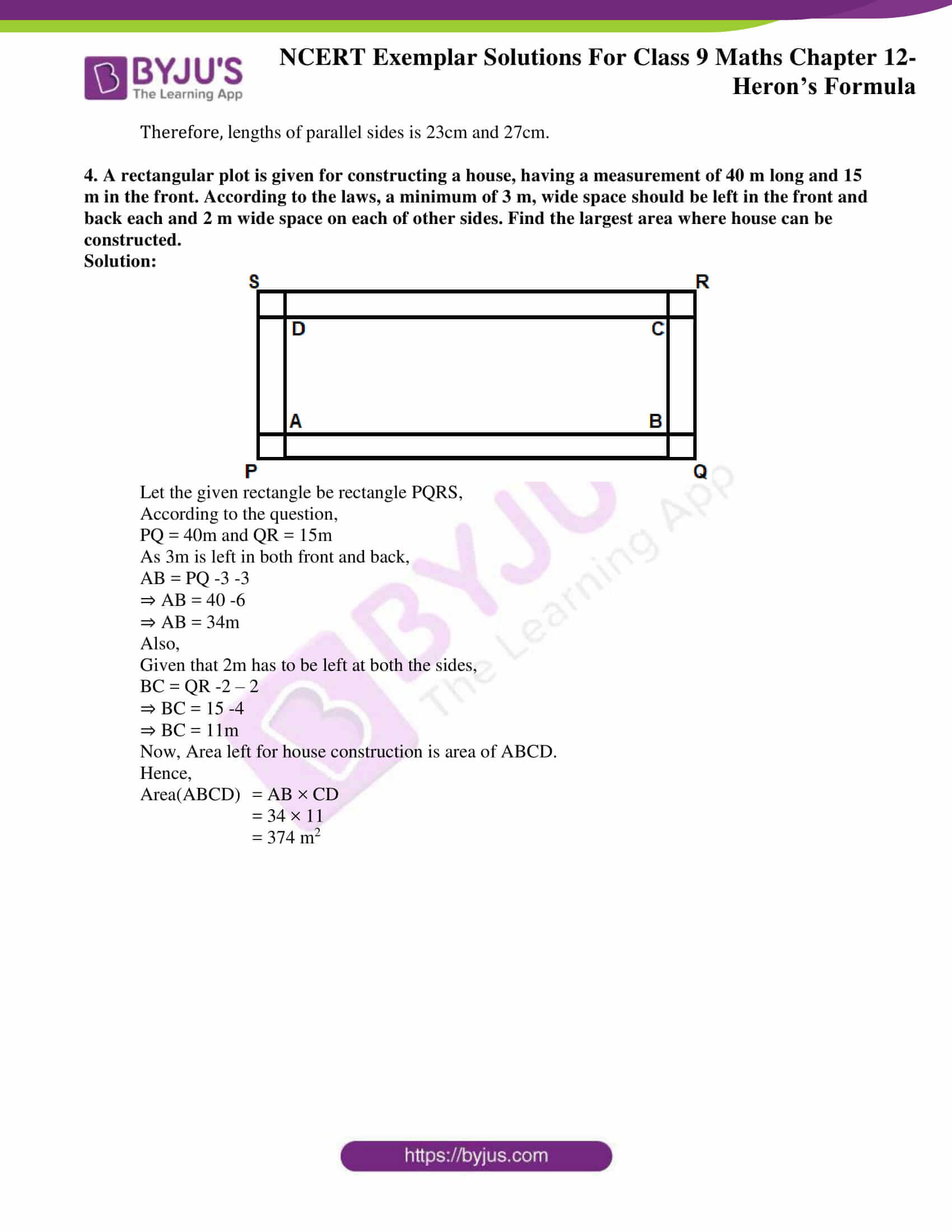### Access Answers to NCERT Exemplar Solutions for Class 9 Maths Chapter 12 Heron’s Formula

Exercise 12.1 Page No: 113

1. An isosceles right triangle has area 8 cm2. The length of its hypotenuse is

(A) √32 cm

(B) √16 cm

(C) √48 cm

(D) √24 cm

Solution:

(A) √32 cm

Explanation:

Let height of triangle = h

As the triangle is isosceles,

Let base = height = h

According to the question,

Area of triangle = 8cm2

⇒ ½ × Base × Height = 8

⇒ ½ × h × h = 8

⇒ h2 = 16

⇒ h = 4cm

Base = Height = 4cm

Since the triangle is right angled,

Hypotenuse2 = Base2 + Height2

⇒ Hypotenuse2 = 42 + 42

⇒ Hypotenuse2 = 32

⇒ Hypotenuse = √32

Hence, Options A is the correct answer.

2. The perimeter of an equilateral triangle is 60 m. The area is

(A) 103 m2

(B) 153 m2

(C) 203 m2

(D) 1003 m2

Solution:

(D) 1003 m2

Explanation:

Area of an equilateral triangle of side a = √3/4 a2

According to the question,

Perimeter of triangle = 60m

⇒ a + a + a = 60

⇒ 3a = 60

⇒ a = 20m

Area of the triangle = (√3/4) a2

= (√3/4) (20)2

= (√3/4) (400)

= 100√3

Hence, Options D is the correct answer.

3. The sides of a triangle are 56 cm, 60 cm and 52 cm long. Then the area of the triangle is

(A) 1322 cm2

(B) 1311 cm2

(C) 1344 cm2

(D) 1392 cm2

Solution:

(C) 1344 cm2

Explanation:

According to the question,

Sides of a triangle,

a = 56, b = 60, c = 52

s = (a + b + c)/2

⇒ s = (56 + 60 + 52)/2

= 168/2 = 84.

Area of triangle = √s(s-a)(s-b)(s-c)

= √84(84-56)(84-60)(84-52)

= √84×28×24×32

= 1344cm2

Hence, Options C is the correct answer.

4. The area of an equilateral triangle with side 23 cm is

(A) 5.196 cm2

(B) 0.866 cm2

(C) 3.496 cm2

(D) 1.732 cm2

Solution:

(A) 5.196 cm2

Explanation:

Area of an equilateral triangle of side a = √3/4 a2

According to the question,

a = 2√3

Area of triangle = (√3/4) a2

= (√3/4) (2√3)2

= (√3/4)(12)

= 3√3

= 5.196

Hence, Options A is the correct answer.

Exercise 12.2 Page No: 115

Write True or False and justify your answer:

1. The area of a triangle with base 4 cm and height 6 cm is 24 cm2.

Solution:

False

Justification:

Area of triangle = ½ × Base × Altitude

= ½ × 4 × 6

= 12cm2

Hence, the statement “the area of a triangle with base 4 cm and height 6 cm is 24 cm2” is False.

2. The area of △ ABC is 8 cm2 in which AB = AC = 4 cm and ∠A = 90º.

Solution:

True

Justification:

Area of triangle = ½ × Base × Altitude

= ½ × 4 × 4

= 8cm2

Hence, the statement is “area of △ABC is 8 cm2 in which AB = AC = 4 cm and ∠A = 90º” is True.

3. The area of the isosceles triangle is 5/4 √11cm2, if the perimeter is 11 cm and the base is 5 cm.

Solution:

True

Justification:

According to the question,

Perimeter = 11cm

And base, a = 5

As the triangle is isosceles, b = c

Perimeter = 11

⇒ a + b + c = 11

⇒ 5 + b + b = 11

⇒ 5 + 2b = 11

⇒ 2b = 6

⇒ b = 3

So, we have,

a = 5, b = 3, c = 3

s = (a + b + c)/2

⇒ s = (5 + 3 + 3)/2 = 11/2

Area of triangle = √s(s-a)(s-b)(s-c)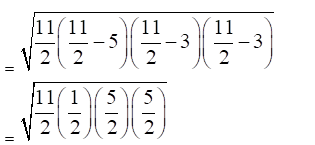⇒ Area of triangle = (5√11)/4cm2

Hence, the statement “The area of the isosceles triangle is 5/4 √11cm2, if the perimeter is 11 cm and the base is 5 cm” is True.

4. The area of the equilateral triangle is 20√3 cm2 whose each side is 8 cm.

Solution:

False

Justification:

Area of an equilateral triangle of side a = √3/4 a2

According to the question,

Area of a triangle = 20√3 cm2

⇒ √3/4 a2 = 20√3

⇒ a2 = 20× 4

⇒ a2 = 80

⇒ a = 4√5 cm

Hence, the statement “the area of the equilateral triangle is 20√3 cm2 whose each side is 8 cm” is False.

Exercise 12.3 Page No: 117

1 Find the cost of laying grass in a triangular field of sides 50 m, 65 m and 65 m at the rate of Rs 7 per m2.

Solution:

According to the question,

Sides of the triangular field are 50 m, 65 m and 65 m.

Cost of laying grass in a triangular field = Rs 7 per m2

Let a = 50, b = 65, c = 65

s = (a + b + c)/2

⇒ s = (50 + 65 + 65)/2

= 180/2

= 90.

Area of triangle = √s(s-a)(s-b)(s-c)

= √90(90-50)(90-65)(90-65)

= √90×40×25×25

= 1500m2

Cost of laying grass = Area of triangle ×Cost per m2

= 1500×7

= Rs.10500

2 The triangular side walls of a flyover have been used for advertisements. The sides of the walls are 13 m, 14 m and 15 m. The advertisements yield an earning of Rs 2000 per m2 a year. A company hired one of its walls for 6 months. How much rent did it pay?

Solution:

According to the question,

The sides of the triangle are 13 m, 14 m and 15 m

Let a = 13, b = 14, c = 15

s = (a + b + c)/2

⇒ s = (13 + 14 + 15)/2

= 42/2

= 21.

Area of triangle = √s(s-a)(s-b)(s-c)

= √21(21-13)(21-14)(21-15)

= √21×8×7×6

= 84m2

Cost of advertisements for a year = Area of triangle × Cost per m2

= 84×2000

= Rs. 168000

Since the board is rented for only 6 months:

Cost of advertisements for 6 months = (6/12) × 168000

= Rs. 84000

3 From a point in the interior of an equilateral triangle, perpendiculars are drawn on the three sides. The lengths of the perpendiculars are 14 cm, 10 cm and 6 cm. Find the area of the triangle.

Solution:

According to the question,

The lengths of the perpendiculars are 14 cm, 10 cm and 6 cm.

We know that,

Area of an equilateral triangle of side a = √3/4 a2

We divide the triangle into three triangles,

Area of triangle = (1/2 × a × 14) + (1/2 × a × 10) + (1/2 × a × 6)

√3/4 a2 = ½ × a × (14 + 10 + 6)

√3/4 a2 = ½ × a × 30

a = 60/√3

= 20√3

Area of the triangle = √3/4 a2

=√3/4 (20√3)2

= 300√3 cm2

4 The perimeter of an isosceles triangle is 32 cm. The ratio of the equal side to its base is 3 : 2. Find the area of the triangle.

Solution:

According to the question,

Perimeter of the isosceles triangle = 32 cm

It is also given that,

Ratio of equal side to base = 3 : 2

Let the equal side = 3x

So, base = 2x

Perimeter of the triangle = 32

⇒ 3x + 3x + 2x = 32

⇒ 8x = 32

⇒ x = 4.

Equal side = 3x = 3×4 = 12

Base = 2x = 2×4 = 8

The sides of the triangle = 12cm, 12cm and 8cm.

Let a = 12, b = 12, c = 8

s = (a + b + c)/2

⇒ s = (12 + 12 + 8)/2

= 32/2

= 16.

Area of the triangle = √s(s-a)(s-b)(s-c)

= √16(16-12)(16-12)(16-8)

= √16×4×4×8

= 32√2 cm2

5 Find the area of a parallelogram given in Fig. 12.2. Also find the length of the altitude from vertex A on the side DC.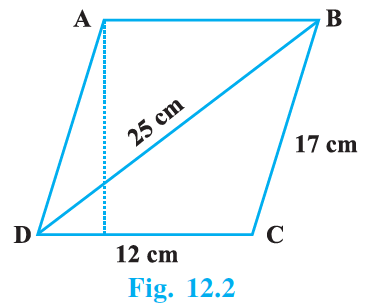Solution:

We know that,

Area of parallelogram(ABCD) = Area(ΔBCD) + Area(ΔABD)

For Area (ΔBCD),

We have,

a = 12, b = 17, c = 25

s = (a + b + c)/2

⇒ s = (12 + 17 + 25)/2 = 54/2 = 27.

Area of (ΔBCD) = √s(s-a)(s-b)(s-c)

= √27(27-12)(27-17)(27-25)

= √27×15×10×2

= 90 cm2

Since, ABCD is a parallelogram,

Area(ΔBCD) = Area(ΔABD)

Area of parallelogram(ABCD) = Area(ΔBCD) + Area(ΔABD)

= 90 + 90

= 180 cm2

Let altitude from A be = x

Also, Area of parallelogram(ABCD) = CD × (Altitude from A)

⇒ 180 = 12 × x

⇒ x = 15 cm

Exercise 12.4 Page No: 118

1. How much paper of each shade is needed to make a kite given in Fig. 12.4, in which ABCD is a square with diagonal 44 cm.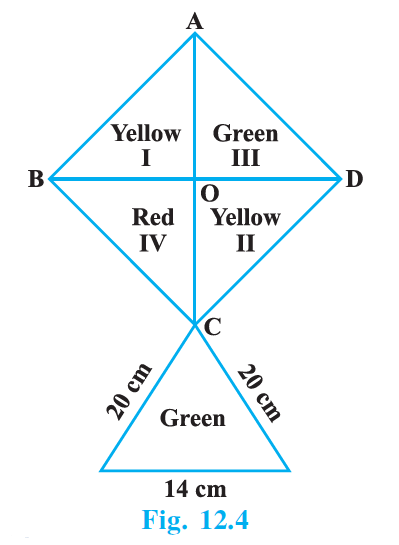Solution:

According to the figure,

AC = BD = 44cm

AO = 44/2 = 22cm

BO = 44/2 = 22cm

From ΔAOB,

AB2 = AO2 + BO2

⇒ AB2 = 222 + 222

⇒ AB2 = 2 × 222

⇒ AB = 22√ 2 cm

Area of square = (Side)2

= (22√2)2

= 968 cm2

Area of each triangle (I, II, III, IV) = Area of square /4

= 968 /4

= 242 cm2

To find area of lower triangle,

Let a = 28, b = 28, c = 14

s = (a + b + c)/2

⇒ s = (28 + 28 + 14)/2 = 70/2 = 35.

Area of the triangle = √s(s-a)(s-b)(s-c)

= √35(35-28)(35-28)(35-14)

= √35×7×7×21

= 49√15 = 189.77cm2

Therefore,

We get,

Area of Red = Area of IV

= 242 cm2

Area of Yellow = Area of I + Area of II

= 242 + 242

= 484 cm2

Area of Green = Area of III + Area of lower triangle

= 242 + 189.77

= 431.77 cm2

2. The perimeter of a triangle is 50 cm. One side of a triangle is 4 cm longer than the smaller side and the third side is 6 cm less than twice the smaller side. Find the area of the triangle.

Solution:

Let the smaller side be = x cm

Then, larger side = (x + 4) cm

And, third side = (2x-6) cm

Given that,

Perimeter = 50 cm

⇒ x + (x + 4) + (2x-6) = 50

⇒ 4x-2 = 50

⇒ 4x = 52

⇒ x = 13

Therefore, smaller side = 13cm

Larger side = x + 4 = 13 + 4 = 17cm

Third side = 2x-6 = 2×13 – 6 = 26-6 = 20cm

To find area of triangle,

Let a = 13, b = 17, c = 20

s = (a + b + c)/2

⇒ s = (13 + 17 + 20)/2 = 50/2 = 25.

Area of triangle = √s(s-a)(s-b)(s-c)

= √25(25-13)(25-17)(25-20)

= √25×12×8×5

= 20√30 cm2

3. The area of a trapezium is 475 cm2 and the height is 19 cm. Find the lengths of its two parallel sides if one side is 4 cm greater than the other.

Solution: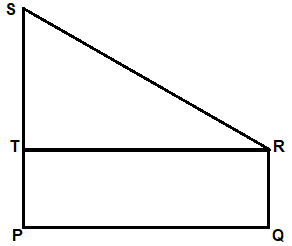Let the given trapezium be PQRS, given in the figure.

According to the question,

PQ = 19cm

Let RQ = x cm

Then,

PS = (x + 4)cm

Construction:

Draw a perpendicular from R on PS which will also be parallel to PQ.

Now,

We get,

PQRT is a rectangle,

Area of rectangle PQRT = PQ × QR

⇒ Area(PQRT) = 19×x = 19x

Now,

PS = PT + TS

Since PT = QR = x cm

(x + 4) = x + TS

⇒ TS = 4cm

Area of triangle RST = ½ × RT × ST

Since RT = PQ = 19cm

⇒ Area(ΔRST) = ½ × 19 × 4

= 38cm2

Area(PQRS) = Area(PQRT) + Area(ΔRST)

⇒ 475 = 19x + 38

⇒ 19x = 475 -38

⇒ 19x = 437

⇒ x = 23 cm

(x + 4) = 23 + 4 = 27cm

Therefore, lengths of parallel sides is 23cm and 27cm.

4. A rectangular plot is given for constructing a house, having a measurement of 40 m long and 15 m in the front. According to the laws, a minimum of 3 m, wide space should be left in the front and back each and 2 m wide space on each of other sides. Find the largest area where house can be constructed.

Solution: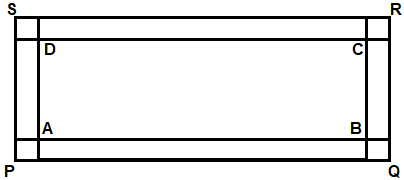Let the given rectangle be rectangle PQRS,

According to the question,

PQ = 40m and QR = 15m

As 3m is left in both front and back,

AB = PQ -3 -3

⇒ AB = 40 -6

⇒ AB = 34m

Also,

Given that 2m has to be left at both the sides,

BC = QR -2 – 2

⇒ BC = 15 -4

⇒ BC = 11m

Now, Area left for house construction is area of ABCD.

Hence,

Area(ABCD) = AB × CD

= 34 × 11

= 374 m2

To provide 9th standard students with more study materials for the exam preparation, BYJU’S is providing notes, exemplar books, question papers and some preparation tips and tricks, so that students can score good marks in Maths subject. They are also suggested to solve sample papers and previous year question papers to get through the idea of question pattern and marking scheme for the chapter, Heron’s formula.

Download BYJU’S -The Learning App and get personalized video content for all the important Maths concepts present in Class 9 and in other classes also. Here, we focus to provide students the best source of learning and make them understand the concepts which are taught in their academics. Keep visiting us and get more updated materials.

## Frequently Asked Questions on NCERT Exemplar Solutions for Class 9 Maths Chapter 12

### Explain the applications of Heron’s formula covered in the Chapter 12 of NCERT Exemplar Solutions for Class 9 Maths.

VHeron’s formula is also applicable to find the area of a quadrilateral if the quadrilaterals are divided into triangular parts. Once the quadrilaterals are divided into triangular forms, apply Heron’s formula to find the area of individual triangular parts. After finding the areas of each triangular part, add all the areas to find the area of quadrilateral.

### Discuss the topics covered in the Chapter 12 of NCERT Exemplar Solutions for Class 9 Maths.

The topics covered in the Chapter 12 of NCERT Exemplar Solutions for Class 9 Maths are –
1. Area of a triangle based on its base and heights
2. Area of a triangle by Heron’s Formula
3. Application of Heron’s Formula in finding areas of Quadrilaterals

### Where can I get the NCERT Exemplar Solutions for Class 9 Maths Chapter 12?

NCERT Exemplar Solutions by BYJU’S is the best reference guide for the students to score well in the annual exam. These solutions contain detailed explanations for each and every concept covered in the chapter. Students who find it difficult in solving the exercise wise problems can access the solutions which are available online to get their doubts cleared instantly. The chapter wise and exercise wise solutions are created by the experts at BYJU’S with the aim of helping students irrespective of their intelligence quotient.